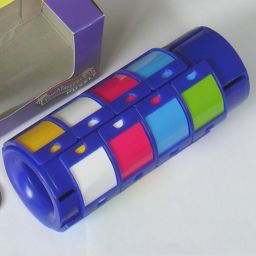# Instant Insanity IIThe Instant Insanity II is a sliding piece tower similar to Missing Link, but with a goal pattern reminiscent of Instant Insanity. It is a cylinder with 16 coloured tiles arranged in four equally spaced columns. The cylinder has five layers, and the top and bottom layers can rotate around the main axis relative to the middle block of three layers. The top layer has only two adjacent spots where a tile can be placed, but all the other layers can contain four tiles.
Each tile has two colours. The face of the tile is of one colour, but there are two small indicator areas on the left and right edges. The right indicator is the same colour as the face of the tile, and the left indicator has a different colour. The aim of the puzzle is to arrange the tiles in the lower four layers so that all four layers and all four columns show four distinct colours, and preferably to do it so that adjacent indicators match in colour.

## The number of positions:

There are 16 distinct pieces and two spaces on a playing board with 18 locations, so that gives a total of 18!/2 = 3,201,186,852,864,000 positions, which are all attainable. There are two essentially unique solutions, but each can have their layers permuted in 4! ways, and the empty top layer can be rotate too, so there are really 2·4!·4 = 192 solved positions amongst the 3.2·1015 total.

## Solution:

There are essentially only two solutions, which are shown below.
 W Y B G Y G R B G B W R B R Y W
 Y G B W W B R G G R Y B B Y W R

It is of course possible to have the layers in a different order, and the orientation of the empty layer does not matter.

Even if you know the colour pattern you want to achieve, there is still the problem of actually manoeuvring the tiles to create that pattern. Assuming that the top layer is the one with only two slots, and that those two slots are empty, then the following move sequences are useful:

A. Move both tiles into the slots of the top layer. Put them back in the layer below but with their positions swapped. Move both tiles from that column into the slots of the top layer Put them back in the column below in the opposite order. Move the whole front column upwards (if necessary, first twist the top layer to bring a slot to the top of the front column). Twist both the top and bottom layers to the left. Move the whole left column downwards. Now apply sequence B to swap the top two tiles of the front column. Move the whole left column upwards. Turn the top and bottom layers to the right. Move the whole front column downwards.

Here is a short description of how to solve it. I will number the layers 1 to 5 from top to bottom.

Phase 1: Solve the bottom layer, layer number 5.

1. Find a tile that you want to put in layer 5.
2. Use the vertical swap sequences B and C above to bring the tile to the layer second from the bottom, layer 4.
3. Turn the bottom layer so that the tile lies directly above the location where you want it to be.
4. Insert the tile by the following sequence:
1. Twist the bottom layer to the left.
2. Move the whole left column upwards (if necessary, first twist the top layer to bring a slot to the top of the left column).
3. Turn the bottom layer to the right.
4. Move the whole front column downwards.
5. Repeat steps a-d until the bottom layer is solved.

Phase 2: Solve the remaining layers

1. First find any tile that belongs in layer 4 but which is incorrectly placed.
2. Bring the tile to layer 2, using if necessary the vertical swap sequences B and C.
3. Use the horizontal swap sequence A above to bring the tile to the correct column.
4. Use the vertical swap sequences B and C above to bring the tile its correct position to layer 4.
5. Repeat a-d until layer 4 is solved.
6. Find any tile that belongs in layer 3 but which is incorrectly placed.
7. Bring the tile to layer 2, using if necessary the vertical swap sequences B.
8. Use the horizontal swap sequence A above to bring the tile to the correct column.
9. Use the vertical swap sequences B above to bring the tile its correct position to layer 3.
10. Repeat f-i until layer 3 is solved.
11. Use the horizontal swap sequence A above put the tiles in layer 2 in the correct locations.